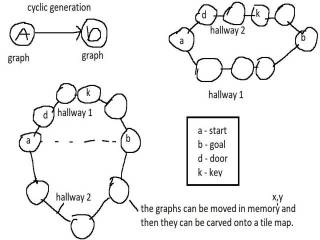-=+=- -=+=- -=+=- -=+=- -=+=- -=+=- -=+=- -=+=- -=+=- -=+=- -=+=- -=+=- -=+=- -=+=- -=+=- -=+=- -=+=- -=+=- -=+=- -=+=- -=+=- -=+=- -=+=- -=+=- -=+=- -=+=- -=+=- -=+=- -=+=- -=+=- (c) WidthPadding Industries 1987 0|319|0 -=+=- -=+=- -=+=- -=+=- -=+=- -=+=- -=+=- -=+=- -=+=- -=+=- -=+=- -=+=- -=+=- -=+=- -=+=- -=+=- -=+=- -=+=- -=+=- -=+=- -=+=- -=+=- -=+=- -=+=- -=+=- -=+=- -=+=- -=+=- -=+=- -=+=-
SoCoder -> Snippet Home -> Generators

PakzCreated : 30 August 2017

Language : Monkey-X

### Cyclic Map Generator

Generate maps where you travel/explore in a circle

Screenshots## Latest Update

I got this from the book : Procedural Generation in game design.

In the book they used graphs for showing how the generation is done and this is something I had not though about using yet. It makes thinking about map generating easier(for me anyway)

The code example here shows how you can generate maps where you can travel towards a destination through a tunnel and then travel back to the start from another tunnel.

I added a image showing how a very basic version of a cyclic generator is designed.

The generator from the code here is also a very basic version. Only a circular path from start to destination and back to start.

Wednesday, 30 August 2017, 04:41
JayenkaiNeat.
With a few offshoot cycles added in, to add complexity to the map, along with some simpler "branches" you could make a really complicated dungeon/maze.Wednesday, 30 August 2017, 04:50
Pakzindeed. I should have known about this way of doing things years ago. The book covers more complex features like you mentioned.

Game design books have been added to my future things to look at list.